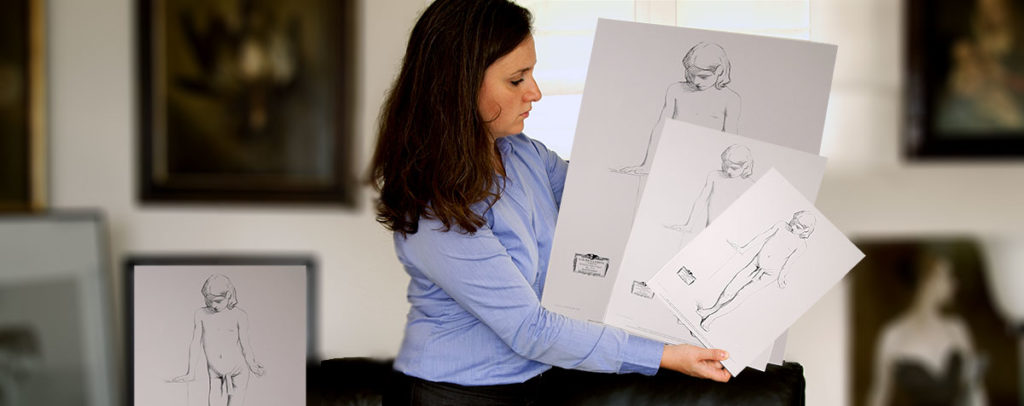## Plates Sizes

The plates are in the first place reproduced at their original size. For Charles Bargue Drawing Course the actual size is 17.32 in X 23.62 in
It’s the size shown on the  photo.
We can produce any size you wish by order. We need up to 5 or 6 weeks to provide the plates. We always try to reduce this delay to give you satisfaction as soon as possible.

## Conversion table inches Centimeters

Inches /Centimeters
in      =   cm

0.39      =     1

1.97   =     5

3.93   =   10

4.33   =   11

4.72   =   12

5.11   =   13

5.51   =   14

5.90   =   15

6.29   =   16

Note : All lithographs are trimed by hand and their sizes may vary a little when paper is cut. It also depends on the papers used for each plate.

Some typical sizes

The first one below is the actual Bargue Drawing Course plates size

17.32 in X 23.62 in   =   44cm X 60cm

11.81 in X 15.74 in         =    30cm X 40cm

7.87 in X 11.81 in           =     20cm X 30cm

Inches / Centimeters

in      =   cm

6.69   =   17

7.08   =   18

7.48   =   19

7.87   =   20

11.81   =30

15.8   =40